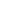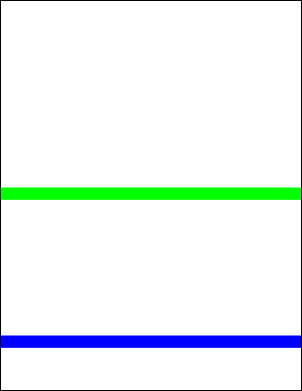AddLine FunctionAdds a line to the current page.Syntax

 [C#] ```int AddLine(double x1, double y1, double x2, double y2) int AddLine(double x1, double y1, double x2, double y2, LineEnding ending1, LineEnding ending2) int AddLine(double x1, double y1, double x2, double y2, LineEnding ending1, LineEnding ending2, double scale1, double scale2)``` [Visual Basic] ```Function AddLine(x1 As Double, y1 As Double, x2 As Double, y2 As Double) As Integer Function AddLine(x1 As Double, y1 As Double, x2 As Double, y2 As Double, ending1 as LineEnding, ending2 as LineEnding) As Integer Function AddLine(x1 As Double, y1 As Double, x2 As Double, y2 As Double, ending1 as LineEnding, ending2 as LineEnding, scale1 As Double, scale2 As Double) As Integer```Params

 Name Description x1 The horizontal offset of the start point. x2 The horizontal offset of the end point. y1 The vertical offset of the start point. y2 The vertical offset of the end point. ending1 The line ending to be placed at the start of the line. Default is None. ending2 The line ending to be placed at the end of the line. Default is None. scale1 The scaling to be applied to the line ending at the start of the line. Default is 1.0. scale2 The scaling to be applied to the line ending at the end of the line. Default is 1.0. return The Object ID of the newly added Graphic Object.Notes

 Adds a line to the current page. The line is drawn in the current color at the current width and with the current options. In addition to the line, you can also specify line endings such as arrows. These can be placed at at the start of the line, the end of the line or both. The LineEnding enumeration may take the following values: None - No symbol Square - A square block Circle - A filled circle Diamond - A filled diamond OpenArrow - Two lines forming an arrow ClosedArrow - A triangular arrow Butt - A perpendicular line BigButt - A longer perpendicular line ReverseOpenArrow - The same as OpenArrow, but pointing backwards rather than forwards ReverseClosedArrow - The same as ClosedArrow, but pointing backwards rather than forwards Slash - A 30 degree line Arrowhead - A barbed arrowhead The size of the line ending is proportional to the width of the line, but you can provide a further scaling factor to make it bigger or smaller. The tip of each line ending is placed at the end of the line so that they can be used for precise positioning. Reversed line endings have a reversed direction, so while the tip is still at the end of the line, the body of the symbol will extend past the end of the line. The AddLine function returns the Object ID of the newly added Graphic Object.Example

 The following code adds two horizontal lines to a document. The first is blue and the second is green.   ```using (Doc doc = new Doc()) {   doc.Width = 24;   doc.Color.String = "0 0 255";   doc.AddLine(-50, 100, 999, 100);   doc.Color.String = "0 255 0";   doc.AddLine(-50, 400, 999, 400);   doc.Save(Server.MapPath("docaddline.pdf")); } ``` ```Using doc As New Doc()   doc.Width = 24   doc.Color.String = "0 0 255"   doc.AddLine(-50, 100, 999, 100)   doc.Color.String = "0 255 0"   doc.AddLine(-50, 400, 999, 400)   doc.Save(Server.MapPath("docaddline.pdf")) End Using ```docaddline.pdf